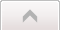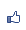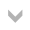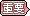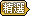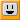[Excel VBA] 身份證字號驗證程式
2009/05/21 19:03:36瀏覽12332｜回應1｜推薦4

(1)第一個字元為地區碼，代表最初登記戶籍的地區，如台北市為 A, 台中市為B,......

 A=10 台北市 J=18 新竹縣 S=26 高雄縣 B=11 台中市 K=19 苗栗縣 T=27 屏東縣 C=12 基隆市 L=20 台中縣 U=28 花蓮縣 D=13 台南市 M=21 南投縣 V=29 台東縣 E=14 高雄市 N=22 彰化縣 W=32 金門縣 F=15 台北縣 O=35 新竹市 X=30 澎湖縣 G=16 宜蘭縣 P=23 雲林縣 Y=31 陽明山 H=17 桃園縣 Q=24 嘉義縣 Z=33 連江縣 I=34 嘉義市 R=25 台南縣

(2)第二個字元性別碼，代表此人的性別，1代表男性，2代表女性。
(3)第三個字元到第九個字元為流水號碼
(4)第十個字元為檢查碼

1. 將地區碼轉換為相對代號，例如：A轉換成10兩個字元，B轉換成11……
對照如下：

 字母 A B C D E F G H I J K L M N O P Q R S T U V W X Y Z 代號 10 11 12 13 14 15 16 17 34 18 19 20 21 22 35 23 24 25 26 27 28 29 32 30 31 33

(需特別注意 I 、O 和 W 的代號)

2.將各數字乘上權數：

(1)英文字母轉成的數字, 個位數乘９再加上十位數
(2)第2~9個字元各數字從右到左依次乘以1、2、3、4......8

3.將乘上權數後之積相加 ：

 字元 A １ ２ ３ ４ ５ ６ ７ ８ 代碼值 1 0 1 2 3 4 5 6 7 8 權數 x1 x9 x8 x7 x6 x5 x4 x3 x2 x1 乘積 1 0 8 14 18 20 20 18 14 8

1 + 0 + 8 + 14 + 18 + 20 + 20 + 18 + 8 = 121

4.相加後之值除以10取其餘數  --

若餘數不為0：由 10 減去餘數得檢查號碼

若餘數為0：檢查碼為 0

121 / 10 = 10 ......1

10 - 1 = 9

Sub test()
If Not CheckTwId("A123456780", ErrorMessage) Then
MsgBox ("身份證字號不正確：" & ErrorMessage)
End If
End Sub

Public Function CheckTwId(ByRef strInput As String, ByRef ErrMsg) As Boolean
Dim strArea, strCID As String
Dim intArea, intCheckNum, intNumSum As Integer
Dim boolValidID As Boolean

boolValidID = True
If Len(strInput) <> 10 Then

Rem 字串長度必須為 10
ErrMsg = "長度不正確" & vbCrLf
boolValidID = False
Else
strFirst = Ucase(Mid(strInput, 1, 1))
Rem 取第一個字元為地區碼, 把小寫轉成大寫

intArea = InStr(1, "ABCDEFGHJKLMNPQRSTUVXYWZIO", strFirst, 1)
Rem 把字母轉換成 1~26 的數字,

If intArea = 0 Then

Rem 若第一個字元不是字母則 intArea 會是 0

ErrMsg = ErrMsg + "第 1 個字元非字母" & vbCrLf
boolValidID = False

End If
For i = 2 To 10

Rem 檢查第 2~10 個字元是否皆為數字
If InStr(1, "0123456789", Mid(strInput, i, 1), 1) = 0 Then
ErrMsg = ErrMsg + "第 " & i & " 個字元非數字" & vbCrLf
boolValidID = False
End If
Next i

Rem 檢查第 2 個字元是否為 1 或 2
If InStr(1, "12", Mid(strInput, 2, 1), 1) = 0 Then
ErrMsg = ErrMsg + "第 2 個字元非1或2" & vbCrLf
boolValidID = False
End If
End If

If boolValidID Then
intCheckNum = CInt(Right(strInput, 1))
Rem 取檢查碼來與計算結果比較
strCID = Format(intArea + 9) & Mid(strInput, 2, 8)

Rem 組成代碼值字串

intNumSum = 0
intNumSum = intNumSum + CInt(Mid(strCID, 1, 1))

Rem 左端第一碼乘以1(可免)
For i = 2 To 10

Rem 第2~10碼依序乘以 9~1 的權數並且加總起來

intNumSum = intNumSum + CInt(Mid(strCID, i, 1)) * (11 - i)
Next i
If (intNumSum Mod 10) = 0 Then

Rem 若除法計算出的餘數為 0 則碼檢核應為 0

If (intCheckNum <> 0) Then
boolValidID = False
ErrMsg = "檢查碼檢核不正確"

End If
Else

If (10 - (intNumSum Mod 10))  <> intCheckNum Then
ErrMsg = "檢查碼檢核不正確"
boolValidID = False
End If
End If
End If
CheckTwId = boolValidID

Rem 傳回表示結果的邏輯值
End Function

( 興趣嗜好電腦3C )引用網址：http://classic-blog.udn.com/article/trackback.jsp?uid=WayCheng&aid=2970149回應文章 選擇排序方式 　最新發表 　最舊發表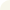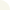Guest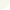A211000000 判斷為錯誤????
2010/05/25 14:24
If (intNumSum Mod 10) = 0 Then '若除法計算出的餘數為 0 則
boolValidID = False
ErrMsg = "檢查碼檢核不正確"
??????????
 ThisIsTheWay(WayCheng) 於 2018-12-24 19:31 回覆： 謝謝您的指正！很抱歉，我今天才發現您的留言。該處程式確實有誤，可能是編輯文章的時候誤刪了 (包括該處的註解也有一半不見了)，剛才已經更正，再次致謝！ Way Cheng 謹致 (2018/12/24)